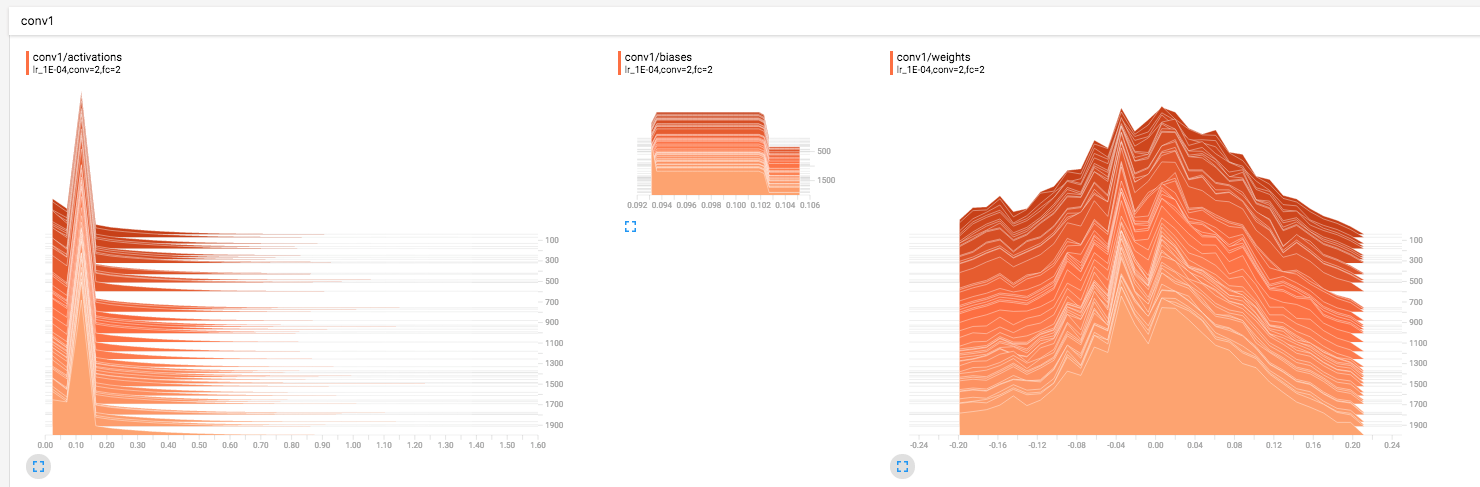Published:

This post shows how to setup tensorboard summaries with popular CNN architecture layers in TF. This does not only help debug but also provide insights into working of deep neural nets.

## Intro

TF has several wrappers such as keras, tf.contrib.slim etc. This is not to replace them, but some helper function to write code faster in TF only environments and also to fully utilize Tensorboard(from TF-dev summit Feb,2017).

# combine all summaries
summ = tf.summary.merge_all()

# write summaries to file
writer = tf.summary.FileWriter(filename)

# when run session
...
...,s = sess.run([..., summ], feed_dict=...)


## Conv Layer

Use this to write a convolution layer with name space. It is very useful for plotting graphs in tensorboard. Modify speciific parameters according to use. This will write summary for weights, biases and activations. These can be visualized using Tensorboard

def conv_layer(input, size_in, size_out,kernel_size,stride=1, layer_name="conv"):
with tf.name_scope(layer_name):
w = tf.Variable(tf.truncated_normal([kernel_size, kernel_size, size_in, size_out], stddev=0.1), name="W")
b = tf.Variable(tf.constant(0.1, shape=[size_out]), name="B")
conv = tf.nn.conv2d(input, w, strides=[1, stride, stride, 1], padding="SAME")
act = tf.nn.relu(conv + b)
tf.summary.histogram("weights", w)
tf.summary.histogram("biases", b)
tf.summary.histogram("activations", act)
return act


## FC Layer

Fully Connected layer

def fc_layer(input, size_in, size_out, layer_name="fc"):
with tf.name_scope(layer_name):
w = tf.Variable(tf.truncated_normal([size_in, size_out], stddev=0.1), name="W")
b = tf.Variable(tf.constant(0.1, shape=[size_out]), name="B")
act = tf.nn.relu(tf.matmul(input, w) + b)
tf.summary.histogram("weights", w)
tf.summary.histogram("biases", b)
tf.summary.histogram("activations", act)
return act


## Batch Norm

Batch-Normalization Layer with trainable parameters.

def batch_norm_layer(input,size_out,layer_name = 'bn'):
with tf.name_scope(layer_name):
beta = tf.Variable(tf.constant(0.0, shape = [size_out]),name = 'beta', trainable = True)
gamma = tf.Variable(tf.constant(1.0, shape = [size_out]),name = 'gamma', trainable = True)
mean, variance = tf.nn.moments(input, [0, 1, 2])
tf.summary.histogram("beta", beta)
tf.summary.histogram("gamma", gamma)
tf.summary.histogram("mean", mean)
tf.summary.histogram("var", variance)
return tf.nn.batch_normalization(input, mean, variance, beta, gamma, variance_epsilon=0.0001, name = 'op')


## Deconvolution

This is required in various VAE or GANs style models

def deconv_layer(input, size_in, size_out,kernel_size,stride=1, layer_name="deconv"):
with tf.name_scope(layer_name):
w = tf.Variable(tf.truncated_normal([kernel_size, kernel_size, size_in, size_out], stddev=0.1), name="W")
b = tf.Variable(tf.constant(0.1, shape=[size_out]), name="B")
conv = tf.nn.conv2d_transpose(input, w, strides=[1, stride, stride, 1], padding="SAME")
act = tf.nn.relu(conv + b)
tf.summary.histogram("weights", w)
tf.summary.histogram("biases", b)
tf.summary.histogram("activations", act)
return act


## Tensorboard OutputTags: